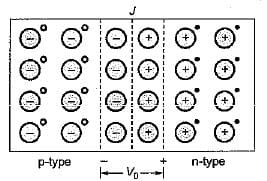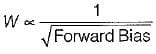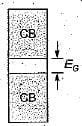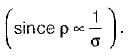Test: Semiconductor Diodes

# Test: Semiconductor Diodes

Test Description

## 10 Questions MCQ Test GATE Electrical Engineering (EE) 2023 Mock Test Series | Test: Semiconductor Diodes

Test: Semiconductor Diodes for Electrical Engineering (EE) 2022 is part of GATE Electrical Engineering (EE) 2023 Mock Test Series preparation. The Test: Semiconductor Diodes questions and answers have been prepared according to the Electrical Engineering (EE) exam syllabus.The Test: Semiconductor Diodes MCQs are made for Electrical Engineering (EE) 2022 Exam. Find important definitions, questions, notes, meanings, examples, exercises, MCQs and online tests for Test: Semiconductor Diodes below.
Solutions of Test: Semiconductor Diodes questions in English are available as part of our GATE Electrical Engineering (EE) 2023 Mock Test Series for Electrical Engineering (EE) & Test: Semiconductor Diodes solutions in Hindi for GATE Electrical Engineering (EE) 2023 Mock Test Series course. Download more important topics, notes, lectures and mock test series for Electrical Engineering (EE) Exam by signing up for free. Attempt Test: Semiconductor Diodes | 10 questions in 30 minutes | Mock test for Electrical Engineering (EE) preparation | Free important questions MCQ to study GATE Electrical Engineering (EE) 2023 Mock Test Series for Electrical Engineering (EE) Exam | Download free PDF with solutions
 1 Crore+ students have signed up on EduRev. Have you?
Test: Semiconductor Diodes - Question 1

### The barrier voltage (V0 or Vr) in a junction diode is the effect of

Detailed Solution for Test: Semiconductor Diodes - Question 1Due to the density gradient across the junction, holes will initially diffuse to the right across the junction, and electrons to the left. The positive holes which neutralizes the acceptor ions near the junction in the p-type silicon disappears as a result of combination with electrons which have diffused across the junction. Similarly, electrons in n-type semiconductor combines with holes.

Test: Semiconductor Diodes - Question 2

### In a zener diode large reverse current is due to

Detailed Solution for Test: Semiconductor Diodes - Question 2

The large reverse current flow in zener diode is due to rupturing-off or tearing-off of covalent bends within the depletion layer. This phenomena is called zener breakdown phenomena, It is due to large electric field intensity.

Test: Semiconductor Diodes - Question 3

### For thermionic emission

Detailed Solution for Test: Semiconductor Diodes - Question 3

The work function of a metal represents the minimum amount of energy that must be given to the fastest-moving electron at the absolute zero of temperature in order for this electron to be able to escape from the metal. Hence, low work function (Ew) is required for thermionic emission.

Test: Semiconductor Diodes - Question 4

The most commonly used type of electron emission in electron tubes is

Test: Semiconductor Diodes - Question 5

When forward bias is applied to a junction diode, it

Detailed Solution for Test: Semiconductor Diodes - Question 5

Width of depletion layer,Hence, when forward bias is applied to a p-n junction diode, width of the depletion layer is reduced i.e. potential barrier is decreased

Test: Semiconductor Diodes - Question 6

The emission of electrons in a vacuum diode is achieved by

Test: Semiconductor Diodes - Question 7

The main reason why electrons can tunnel through a p-n junction is that

Detailed Solution for Test: Semiconductor Diodes - Question 7

In a p-n junction tunnel diode, the depletion layer width is negligible and therefore, the charge carriers will be penetrating the depletion layer almost at the speed of light and this property is called tunnelling of charge carriers across the junction.

Test: Semiconductor Diodes - Question 8

The forbidden energy gap in semiconductors

Detailed Solution for Test: Semiconductor Diodes - Question 8

The energy level diagram for a semiconductor is shown below.EG is called “band gap” or "forbidden energy gap” which lies between the valence band and the conduction band.

Test: Semiconductor Diodes - Question 9

The barrier potential for a Ge p-n junction is

Detailed Solution for Test: Semiconductor Diodes - Question 9

Typical value of “Barrier voltage” or "Contact potential” for a p-n junction is:
V0 = 0.2 V (for Ge p-n junction diode)
= 0.7V (for Si p-n junction diode)

Test: Semiconductor Diodes - Question 10

With the increase in temperature, the resistivity of an intrinsic semiconductor decreases. This is because, with the increase of temperature

Detailed Solution for Test: Semiconductor Diodes - Question 10

In an intrinsic semiconductor, as the temperature increases mobility is slightly reduced since mobility of charge carrier is inversely proportional to temperature. This will slightly reduce the conductivity. But, due to temperature effect, large number of covalent bonds are broken which creates electrons and holes and this increases the conductivity by a larger value. As a result of this resistivity is decreased## GATE Electrical Engineering (EE) 2023 Mock Test Series

18 docs|208 tests
 Use Code STAYHOME200 and get INR 200 additional OFF Use Coupon Code
Information about Test: Semiconductor Diodes Page
In this test you can find the Exam questions for Test: Semiconductor Diodes solved & explained in the simplest way possible. Besides giving Questions and answers for Test: Semiconductor Diodes, EduRev gives you an ample number of Online tests for practice

## GATE Electrical Engineering (EE) 2023 Mock Test Series

18 docs|208 tests